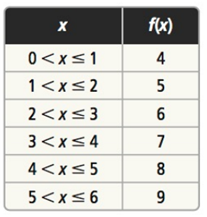Mathematics
Easy

Question

# For the following table, write a rule for f using the ceiling or floor function## f(x) = floor (x) + 4 , 0 ≤ x ≤ 6 f(x) = floor (x) + 3 , 0 < x ≤ 6 f(x) = ceiling (x) + 4 , 0 < x < 6 f(x) = ceiling (x) + 3 , 0 < x ≤ 6Hint:

## The correct answer is: f(x) = ceiling (x) + 3 , 0 < x ≤ 6

### Related Questions to study#### With Turito Foundation.#### Get an Expert Advice From Turito.